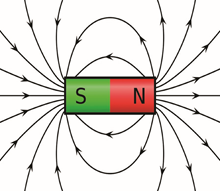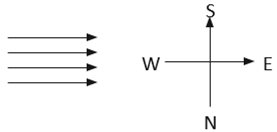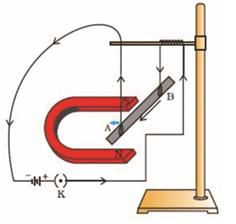# NCERT Class 10: Science-Intext Solutions

### Chapter 13-Magnetic Effects of Electric Current-Intext Solutions

NCERT Book Page Number-224
Q.1. Why does a compass needle get deflected when brought near a bar magnet?
Ans. A compass needle is a small bar magnet. When it is brought near a bar magnet, its magnetic field lines interact with that of the bar magnet. Hence, a compass needle shows a deflection when brought near the bar magnet.

NCERT Book Page Number-228

Q.1. Draw magnetic field lines around a bar magnet.
Ans. Magnetic field lines of a bar magnet emerge from the north pole and terminate at the south pole. Inside the magnet, the field lines emerge from the south pole and terminate at the north pole, as shown in the given figure.Q.2. List the properties of magnetic lines of force.
Ans. The properties of magnetic lines of force are as follows :
(a) Magnetic field lines emerge from the north pole.
(b) They merge at the south pole.
(c) The direction of field lines inside the magnet is from the south pole to the north pole.
(d) Magnetic lines do not intersect with each other.

Q.3. Why don’t two magnetic lines of force intersect each other?
Ans. If two field lines of a magnet intersect, then at the point of intersection, the compass needle would point in two different directions. This is not possible. Hence, two field lines do not intersect each other.

NCERT Book Page Number-229-230

Q.1. Consider a circular loop of wire lying in the plane of the table. Let the current pass through the loop clockwise. Apply the right-hand rule to find out the direction of the magnetic field inside and outside the loop.
Ans. Inside the loop → Pierce into the table
Outside the loop → Appear to emerge out from the table
For clockwise direction of current flowing in the circular loop, the direction of magnetic field lines will be as if they are emerging from the table outside the loop and merging in the table inside the loop.
Q.2. The magnetic field in a given region is uniform. Draw a diagram to represent it.
Ans. A uniform magnetic field means number of field lines are same in the given area, or the field lines are parallel and equispaced to each other. The diagram is given below, where the direction of field is form west in east.Q.3. Choose the correct option.
The magnetic field inside a long straight solenoid-carrying current
(a) is zero
(b) decreases as we move towards its end
(c) increases as we move towards its end
(d) is the same at all points
Ans. (d) The magnetic field inside a long, straight, current-earning solenoid is uniform. It is the same at all points inside the solenoid.

NCERT Book Page Number-231-232

Q. 1. Which of the following property of a proton can change while it moves freely in a magnetic field ? (There may be more than one correct answer.)
(a) mass
(b) speed
(c) velocity
(d) momentum
Ans. (c) and (d)
When a proton enters in a region of magnetic field, it experiences a magnetic force. As a result of the force, the path of the proton becomes circular. Hence, its velocity and momentum change.
Q.2. In the figure, how do you think the displacement of rod AB will be affected if (i) current in rod AB is increased: (ii) a stronger horse-shoe magnet is used: and (iii) length of the rod AB is increased?Ans. A current-carrying conductor placed in a magnetic field experiences a force. The magnitude of force increases with the amount of current, strength of the magnetic field, and the length of the conductor. Hence, the magnetic force exerted on rod AB and its deflection will increase if
(i) current in rod AB is increased
(ii) a stronger horse-shoe magnet is used
(iii) length of rod AB is increased

Q.3. A positively-charged particle (alpha-particle) projected towards west is deflected towards north by a magnetic field. The direction of magnetic field is
(a) towards south
(b) towards east
(c) downward
(d) upward
Ans. (d) The direction of the magnetic field can be determined by the Fleming’s left hand rule. According this rule, if we arrange the thumb, the centre finger, and the forefinger of the left hand at right angles to each other, then the thumb points towards the direction of the magnetic force, the centre finger gives the direction of current, and the forefinger points in the direction of magnetic field. Since the direction of positively charged alpha particle is towards west, the direction of current will be the same i.e., towards west. Again, the direction of magnetic force is towards north. Hence, according to Fleming’s left hand rule, the direction of magnetic field will be upwards.

NCERT Book Page Number-233

Q.1. State Fleming’s left-hand rule.
Ans. Fleming’s left hand rule states that if we arrange the thumb, the centre finger, and the forefinger of the left hand at right angles to each other, then the thumb points towards the direction of the magnetic force, the centre finger gives the direction of current, and the forefinger points in the direction of magnetic field.
Q.2. What is the principle of an electric motor?
Ans. The working principle of an electric motor is based on the magnetic effect of current. A current-carrying loop experiences a force and rotates when placed in a magnetic field. The direction of rotation of the loop is given by the Fleming’s left-hand rule.
Q.3. What is the role of the split ring in an electric motor?
Ans. The split ring in the electric motor acts as a commutator. The commutator reverses the direction of current flowing through the coil after each half rotation of the coil. Due to this reversal of the current, the coil continues to rotate in the same direction.

NCERT Book Page Number-236

Q.1. Explain different ways to induce current in a coil.
Ans. The different ways to induce current in a coil are as follows:
(a) If a coil is moved rapidly between the two poles of a horse-shoe magnet, then an electric current is induced in the coil.
(b) If a magnet is moved relative to a coil, then an electric current is induced in the coil.

NCERT Book Page Number-237

Q.1. State the principle of an electric generator.
Ans. An electric generator works on the principle of electromagnetic induction. It generates electricity by rotating a coil in a magnetic field.
Q.2. Name some sources of direct current.
Ans. Some sources of direct current are electric cell, Solar cell, DC generator, etc.
Q.3. Which sources produce alternating current ?
Ans. AC generators, power plants, etc., produce alternating current.
Q.4. Choose the correct option.
A rectangular coil of copper wires is rotated in a magnetic field. The direction of the induced current changes once in each
(a) two revolutions
(b) one revolution
(c) half revolution
(d) one-fourth revolution
Ans. (c) When a rectangular coil of copper is rotated in a magnetic field, the direction of the induced current in the coil changes once in each half-revolution.

NCERT Book Page Number-238

Q.1. Name two safety measures commonly used in electric circuits and appliances.
Ans. Two safety measures commonly used in electric circuits and appliances are as follows :
(i) Each circuit must be connected with an electric fuse. This prevents the flow of excessive current through the circuit. When the current passing through the wire exceeds the maximum limit of the fuse element, the fuse melts to stop the flow of current through that circuit, hence protecting the appliances connected to the circuit.
(ii) Earthing is a must to prevent electric shocks. Any leakage of current in an electric appliance is transferred to the ground and people using the appliance do not get the shock.
Q.2. An electric oven of 2 kW is operated in a domestic electric circuit (220 V) that has a current rating of 5 A. What result do you expect? Explain.
Ans. Current drawn by the electric oven can be obtained by the expression
P = VI
I = P/V
Where,
Current = I
Power of the oven, P = 2kW = 2000 W
Voltage supplied, V = 220 V
I = 2000 W/ 220 V = 9.09 A
Hence, the current drawn by the electric oven is 9.09 A, which exceeds the safe limit of the circuit. Fuse element of the electric fuse will melt and break the circuit.
Q.3. What precaution should be taken to avoid the overloading of domestic electric circuits?
Ans. The precautions that should be taken to avoid the overloading of domestic circuits are as follows:
(a) Too many appliances should not be connected to a single socket.
(b) Too many appliances should not be used at the same time.
(c) Faulty appliances should not be connected in the circuit.
(d) Fuse should be connected in the circuit.